• 深度学习 deep learning 吴恩达 课后作业 中文版
万次阅读
2019-08-02 20:23:57

深度学习 吴恩达 课后作业 中文版
deep learning

更多相关内容
• YOLO,吴恩达课后编程作业,Course 4 - 卷积，训练model_data/yolov2.h5的项目，直接下载，查看我发的另一篇博客关于这个资源的使用
• 该资源是吴恩达公开课后的8次作业的习题+编程答案，编程答案分为python版本和matlab版本
• 【目录】【吴恩达课后作业目录】 吴恩达深度学习相关资源下载地址（蓝奏云） 课程 周数 名称 类型 语言 地址 课程1 - 神经网络和深度学习 第1周 深度学习简介 测验 中英 传送门 无编程作业 编程作业 —— —— 第2周...
 【目录】【吴恩达课后作业目录】 吴恩达深度学习相关资源下载地址（蓝奏云） 课程 周数 名称 类型 语言 地址 课程1 - 神经网络和深度学习 第1周 深度学习简介 测验 中英 传送门 无编程作业 编程作业 —— —— 第2周 神经网络基础 测验 中英 传送门 具有神经网络思维的Logistic回归 编程作业 中文 传送门 第3周 浅层神经网络 测验 中英 传送门 带有一个隐藏层的平面数据分类 编程作业 中文 传送门 第4周 深度神经网络的关键概念 测验 中英 传送门 一步步搭建多层神经网络以及应用（1 & 2） 编程作业 中文 传送门 课程2 - 改善深层神经网络 第1周 深度学习的实践 测验 中英 传送门 初始化、正则化、梯度校验（1 & 2 & 3） 编程作业 中文 传送门 第2周 优化算法 测验 中英 传送门 优化算法实战 编程作业 中文 传送门 第3周 超参数调整，批量标准化，编程框架 测验 中英 传送门 TensorFlow入门 编程作业 中文 传送门 课程3 - 结构化机器学习项目 第1周 和平之城中的鸟类识别(案例研究) 测验 中英 传送门 无编程作业 编程作业 —— —— 第2周 自动驾驶（案例研究） 测验 中英 传送门 无编程作业 编程作业 —— —— 课程4 - 卷积神经网络 第1周 卷积神经网络的基本知识 测验 中英 传送门 搭建卷积神经网络模型以及应用（1&2） 编程作业 中文 传送门 第2周 深度卷积模型 测验 中英 传送门 Keras入门与残差网络的搭建 编程作业 中文 传送门 第3周 检测算法 测验 中英 传送门 车辆识别 编程作业 中文 传送门 第4周 特殊应用：人脸识别和神经风格转换 测验 中英 传送门 人脸识别与神经风格转换 编程作业 中文 传送门 课程5 - 序列模型 第1周 循环神经网络 测验 中英 传送门 搭建循环神经网络及其应用 编程作业 中文 传送门 第2周 自然语言处理与词嵌入 测验 中英 传送门 词向量的运算与Emoji生成器 编程作业 中文 传送门 第3周 序列模型与注意力机制 测验 中英 传送门 机器翻译与触发词检测 编程作业 中文 传送门 吴恩达深度学习相关资源下载地址（蓝奏云）
展开全文• 吴恩达 深度学习课后作业 深度学习系列课后作业系列1 ，week2课后作业原题 看完视频还是得多多练习。
• ## 最详细吴恩达课后作业Python实现

千次阅读 多人点赞 2021-05-21 20:45:41
ex I-代码实现 单变量线性回归 在本部分的练习中，您将使用...按照老师上课时候所说的来准备数据,取最后一列为目标向量,剩余列为输入矩阵X,最重要的是记得在第一列加入新的一列,数值全部为1 import numpy as np impo

## ex I-代码实现

### 单变量线性回归

在本部分的练习中，您将使用一个变量实现线性回归，以预测食品卡车的利润。假设你是一家餐馆的首席执行官，正在考虑不同的城市开设一个新的分店。该连锁店已经在各个城市拥有卡车，而且你有来自城市的利润和人口数据。

您希望通过使用这些数据来帮助您扩展到下一个城市；

#### Step1: Prepare datasets

按照吴老师上课时候所说的来准备数据,取最后一列为目标向量,剩余列为输入矩阵X,最重要的是记得在第一列加入新的一列,数值全部为1

import numpy as np
import pandas as pd
import matplotlib.pyplot as plt

'''
单变量线性回归
1.Prepare datasets
'''
path = 'ex1data1.txt'
data.describe()     # describe快速统计汇总数据内容
# print(data.describe())
# 展示散点图，可视化理解数据
# data.plot(kind='scatter', x='Population', y='Profit', figsize=(8, 5))
# plt.show()

data.insert(0, 'Ones', 1)       # 加入至第一列，全部数值为1
# print(data)

# 变量初始化:set X (training data) and y (target variable)
cols = data.shape  # 列数
X = data.iloc[:, 0:cols - 1]        # iloc根据位置索引选取数据，先行后列,取前cols-1列作为输入向量
y = data.iloc[:, cols - 1:cols]     # 取最后一列作为目标向量

X = np.matrix(X.values)
y = np.matrix(y.values)
theta = np.matrix([0, 0])
print(X.shape, y.shape, theta.shape)  # 查看各自的行列数


#### Step2: Design model using Gradient Descent

首先, 我们将创建一个以参数 θ \theta 为特征函数的代价函数
J ( θ ) = 1 2 m ∑ i = 1 m ( h θ ( x ( i ) ) − y ( i ) ) 2 J(\theta)=\frac{1}{2 m} \sum_{i=1}^{m}\left(h_{\theta}\left(x^{(i)}\right)-y^{(i)}\right)^{2}
其中: h θ ( x ) = θ T X = θ 0 x 0 + θ 1 x 1 + θ 2 x 2 + … + θ n x n h_{\theta}(x)=\theta^{T} X=\theta_{0} x_{0}+\theta_{1} x_{1}+\theta_{2} x_{2}+\ldots+\theta_{n} x_{n}
计算代价函数 J ( θ ) J(\theta)

'''
'''
def computeCost(X, y, theta):
'''
作用：计算代价函数,向量化来计算参数
:param X: 输入矩阵
:param y: 输出目标
:param theta: parameters
:return:
'''
inner = np.power(((X * theta.T) - y), 2)
# print(inner)
return sum(inner) / (2 * len(X))

# testing
# computeCost(X, y, theta)  # 32.07273388


注意：这里我使用的是matix而不是array，两者基本通用。

但是matrix的优势就是相对简单的运算符号，比如两个矩阵相乘，就是用符号*，但是array相乘不能这么用，得用方法.dot()
array的优势就是不仅仅表示二维，还能表示3、4、5…维，而且在大部分Python程序里，array也是更常用的。

两者区别：

对应元素相乘：matrix可以用np.multiply(X2,X1)，array直接X1X2
点乘：matrix直接X1
X2，array可以 X1@X2 或 X1.dot(X2) 或 np.dot(X1, X2)
代价函数是应该是numpy矩阵，所以我们需要转换X和Y，然后才能使用它们。 我们还需要初始化theta。

优化:
θ j : = θ j − α ∂ ∂ θ j J ( θ ) θ j : = θ j − α 1 m ∑ i = 1 m ( h θ ( x ( i ) ) − y ( i ) ) x j ( i ) \begin{array}{c} \theta_{j}:=\theta_{j}-\alpha \frac{\partial}{\partial \theta_{j}} J(\theta) \\ \theta_{j}:=\theta_{j}-\alpha \frac{1}{m} \sum_{i=1}^{m}\left(h_{\theta}\left(x^{(i)}\right)-y^{(i)}\right) x_{j}^{(i)} \end{array}
使用 vectorization同时更新所有的 θ \theta , 可以大大提高效率

def gradientDescent(X, y, theta, alpha, epoch):
'''
作用:获得最终梯度下降后的theta值以及cost
:param X:
:param y:
:param theta:
:param alpha:
:param epoch:
:return:
'''
# 变量初始化，储存数据
temp = np.matrix(np.zeros(theta.shape))  # 初始化一个临时矩阵(1, 2)
# flatten()即返回一个折叠成一维的数组。但是该函数只能适用于numpy对象，即array或者mat，普通的list列表是不行的。
parameters = int(theta.flatten().shape)  # 参数theta的数量
cost = np.zeros(epoch)  # 初始化一个ndarray, 包含每次训练后的cost
counterTheta = np.zeros((epoch, 2))
m = X.shape  # 样本参数

for i in range(epoch):
'''
使用 vectorization同时更新所有的θ，可以大大提高效率,此处都是相对应的进行计算
X.shape, theta.shape, y.shape, X.shape
((97, 2), (1, 2), (97, 1), 97)
'''
temp = theta - (alpha / m) * (X * theta.T - y).T * X

# 以下是不用Vectorization求解梯度下降
# error = (X * theta.T) - y  # (97, 1)
# for j in range(parameters):
#    term = np.multiply(error, X[:,j])  # (97, 1)
#    temp[0,j] = theta[0,j] - ((alpha / m) * np.sum(term))  # (1,1)

theta = temp
counterTheta[i] = theta
cost[i] = computeCost(X, y, theta)
pass
return counterTheta, theta, cost


#### Step3: Run model and Plot

最后一步就是将准备好的数据输入到相应的模型,得出两个图,第一个图是拟合数据图,第二个图是代价图

'''
3.Run model and Plot
'''

alpha = 0.01
epoch = 3800

counterTheta, final_theta, cost = gradientDescent(X, y, theta, alpha, epoch)
computeCost(X, y, final_theta)

x = np.linspace(data.Population.min(), data.Population.max(), 100)  # xlabel
f = final_theta[0, 0] + (final_theta[0, 1] * x)  # ylabel profit

fig1, ax = plt.subplots(figsize=(6, 4))
ax.plot(x, f, 'r', label='Prediction')
ax.scatter(data.Population, data.Profit, label='Training Data')
ax.legend(loc=2)
ax.set_xlabel('Population')
ax.set_ylabel('Profit')
ax.set_title('Predicted Profit vs. Population Size')

fig2, ax = plt.subplots(figsize=(8, 4))
ax.plot(np.arange(epoch), cost, 'r')
ax.set_xlabel('Iteration')
ax.set_ylabel('Cost')
ax.set_title('Error vs. Training Epoch')
plt.show()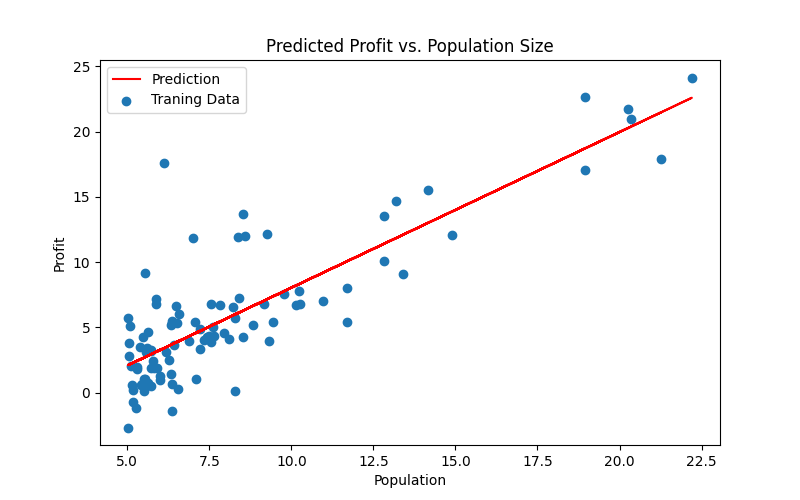可以看出来拟合的相当不错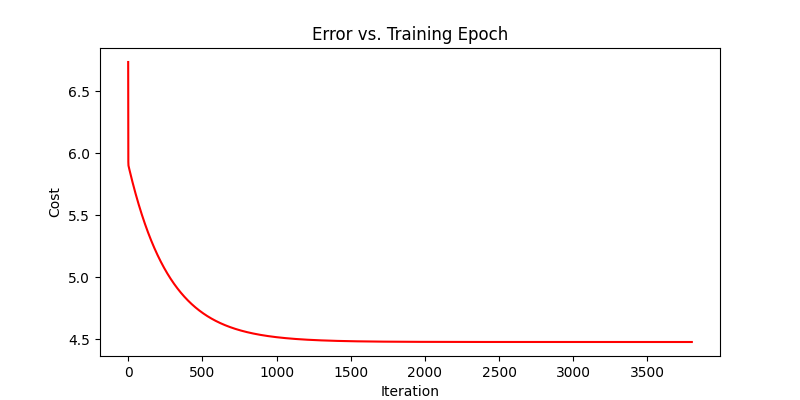最后大概是收敛到4.47左右,如以下图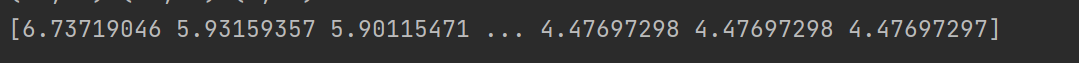#### 使用别的方法同样可以达到以上成果

##### 1.normal equation(正规方程)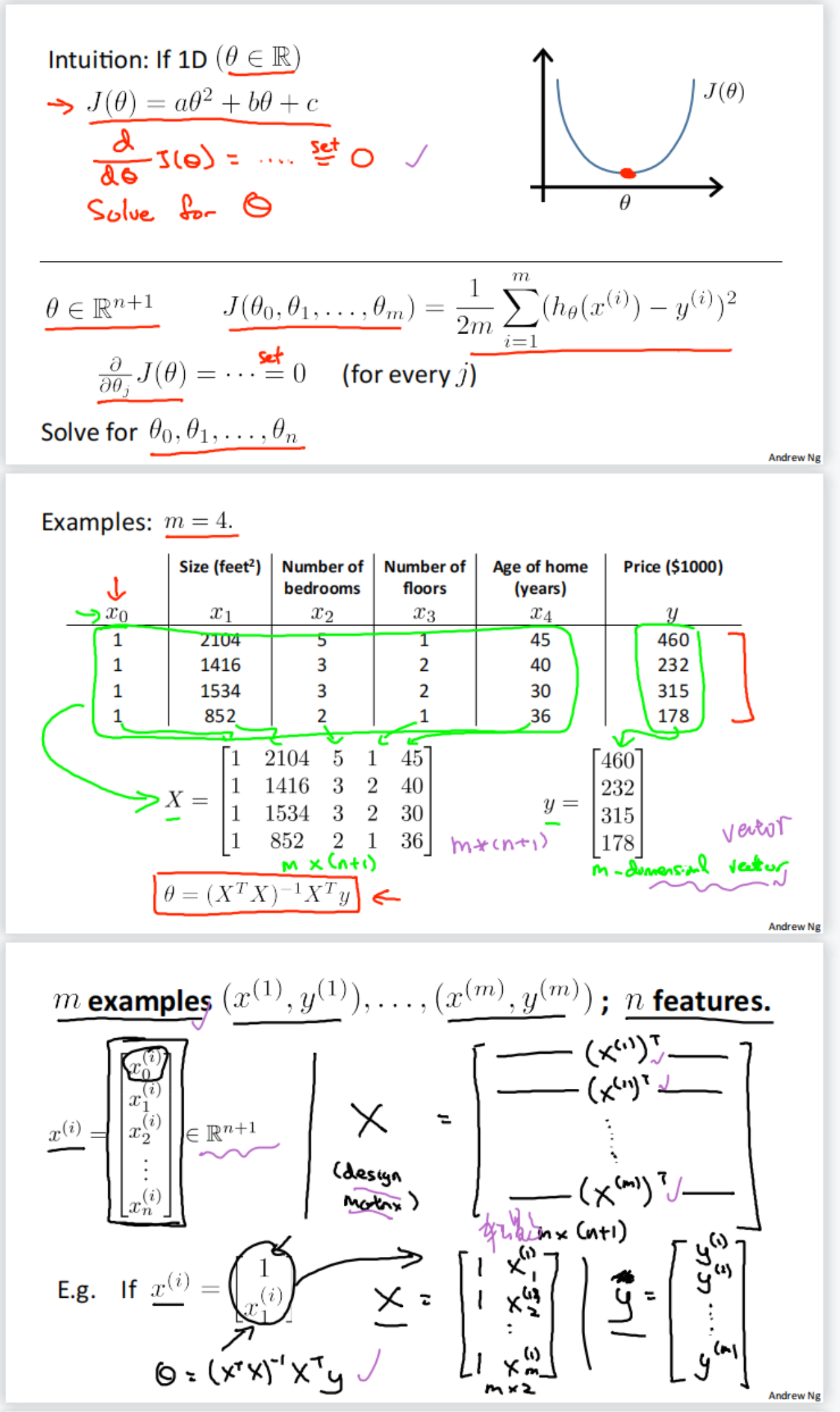梯度下降与正规方程的比较：

梯度下降：需要选择学习率α，需要多次迭代，当特征数量n大时也能较好适用，适用于各种类型的模型

正规方程：不需要选择学习率 α \alpha , 一次计算得出, 需要计算 ( X T X ) − 1 \left(X^{T} X\right)^{-1} , 如果特征数量n较大则运算代 价大，因为矩阵逆的计算时间复杂度为 O ( n 3 ) O(n 3) , 通常来说当 n n 小于10000 时还是可以接受的，只适用 于线性模型，不适合逻辑回归模型等其他模型

def normalEqu(X, y):
theta = np.linalg.inv(X.T@X)@X.T@y      # X.T@X等价于X.T.dot(X)
return theta

final_theta2 = normalEqu(X, y)

x = np.linspace(data.Population.min(), data.Population.max(), 100)  # xlabel
f = final_theta[0, 0] + (final_theta[0, 1] * x)  # ylabel profit

fig3, ax = plt.subplots(figsize=(6, 4))
ax.plot(x, f, 'r', label='Prediction')
ax.scatter(data.Population, data.Profit, label='Training Data')
ax.legend(loc=2)
ax.set_xlabel('Population')
ax.set_ylabel('Profit')
ax.set_title('Predicted Profit vs. Population Size')
plt.show()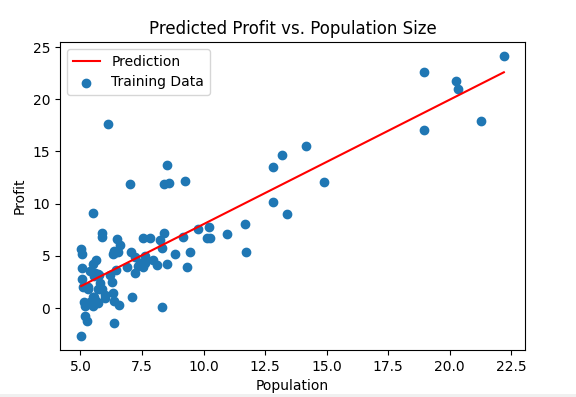##### 调用sklearn库来进行拟合

接着上面代码来

from sklearn import linear_model

'''
2.Design model using sklearn
'''
model = linear_model.LinearRegression()
model.fit(X, y)
# print(X)
# print(X[:, 1].A1.shape)
x = np.array(X[:, 1].A1)    # numpy.matrix[:, 1].A1-->取矩阵的第二列 转换成一维的
f = model.predict(X).flatten()  # 预测之后也转换成一维的

fig, ax = plt.subplots(figsize=(8, 5))
ax.plot(x, f, 'r', label='Prediction')
ax.scatter(data.Population, data.Profit, label='Traning Data')
ax.legend(loc=2)
ax.set_xlabel('Population')
ax.set_ylabel('Profit')
ax.set_title('Predicted Profit vs. Population Size')
plt.show()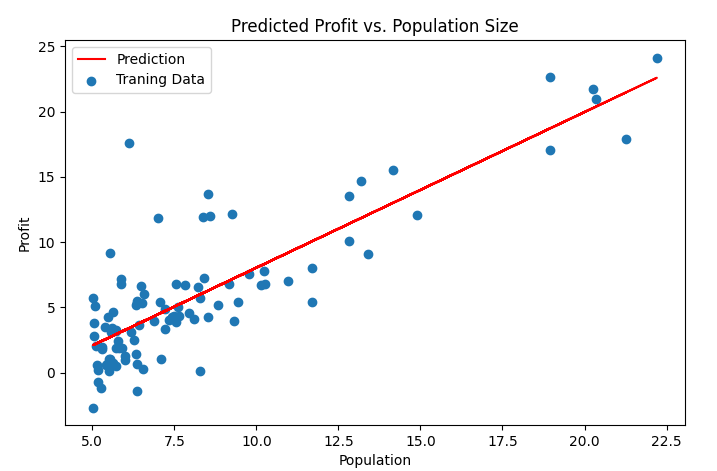### 多向量线性回归

这是ex1中另外一组数据,是多维的数据,其中包括房子的大小,以及卧室的多少,这个时候可以运用到一个小技巧就是特征值归一化

具体的不多说,看代码和图,可直接复制并且运行.

import numpy as np
import pandas as pd
import matplotlib.pyplot as plt

'''
多变量线性回归
1.Prepare datasets
'''
path = 'ex1data2.txt'
# 预处理-特征值归一化
data = (data - data.mean())/data.std()      # std为标准差
data.insert(0, 'Ones', 1)

# set X (training data) and y (target variable)
cols = data.shape
X = data.iloc[:, 0:cols - 1]
y = data.iloc[:, cols - 1:cols]

# convert to matrices and initialize theta
X = np.matrix(X.values)
y = np.matrix(y.values)
theta = np.matrix(np.array([0, 0, 0]))

'''
'''

def computeCost(X, y, theta):
'''
作用：计算代价函数,向量化来计算参数
:param X: 输入矩阵
:param y: 输出目标
:param theta: parameters
:return:
'''
inner = np.power(((X * theta.T) - y), 2)
# print(inner)
return sum(inner) / (2 * len(X))

# testing
# computeCost(X, y, theta)  # 32.07273388

def gradientDescent(X, y, theta, alpha, epoch):
'''
作用:获得最终梯度下降后的theta值以及cost
:param X:
:param y:
:param theta:
:param alpha:
:param epoch:
:return:
'''
# 变量初始化，储存数据
temp = np.matrix(np.zeros(theta.shape))  # 初始化一个临时矩阵(1, 2)
# flatten()即返回一个折叠成一维的数组。但是该函数只能适用于numpy对象，即array或者mat，普通的list列表是不行的。
parameters = int(theta.flatten().shape)  # 参数theta的数量
cost = np.zeros(epoch)  # 初始化一个ndarray, 包含每次训练后的cost
counterTheta = np.zeros((epoch, 3))
m = X.shape  # 样本参数

for i in range(epoch):
'''
使用 vectorization同时更新所有的θ，可以大大提高效率,此处都是相对应的进行计算
X.shape, theta.shape, y.shape, X.shape
((97, 2), (1, 2), (97, 1), 97)
'''
temp = theta - (alpha / m) * (X * theta.T - y).T * X

# 以下是不用Vectorization求解梯度下降
# error = (X * theta.T) - y  # (97, 1)
# for j in range(parameters):
#    term = np.multiply(error, X[:,j])  # (97, 1)
#    temp[0,j] = theta[0,j] - ((alpha / m) * np.sum(term))  # (1,1)

theta = temp
counterTheta[i] = theta
cost[i] = computeCost(X, y, theta)
pass
return counterTheta, theta, cost

'''
3.Run model and Plot
'''

alpha = 0.01
epoch = 3800

counterTheta, final_theta, cost = gradientDescent(X, y, theta, alpha, epoch)
computeCost(X, y, final_theta)

fig2, ax = plt.subplots(figsize=(8, 4))
ax.plot(np.arange(epoch), cost, 'r')
ax.set_xlabel('Iteration')
ax.set_ylabel('Cost')
ax.set_title('Error vs. Training Epoch')
plt.show()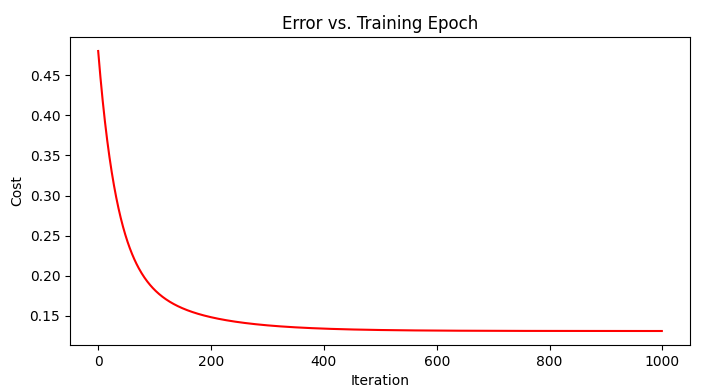参考链接:
https://blog.csdn.net/Cowry5/article/details/80174130

展开全文python 机器学习 人工智能
• csdn上一些吴恩达机器学习作业数据集都是需要积分下载，我在其他网站收集了所有的作业以及数据集，帮助大家学习和交流。
• 【超详细】【吴恩达课后作业】Course 1-神经网络与深度学习-第四周编程作业-附件资源
• 目录0、导入包1、数据预处理2、前向传播——计算梯度和损失3、优化函数——梯度下降4、预测函数5、Logistic回归in神经网络6、main函数7、运行结果8、补充 0、导入包 h5py：因为数据集是H5类型的文件，需要导入包使用...
• 吴恩达课后编程作业】Course+1+-+神经网络和深度学习+-+第四周作业(1&2).zip
• 包含lr_utils.py，train_catvnoncat.h5，test_catvnoncat.h5和示例代码，可直接运行
• 吴恩达老师的机器学习作业内有答案python代码,非常详细，适合新手入门使用。作业可以配合视频一起使用。机器学习
• 吴恩达课后编程作业】Course 2 - 改善深层神经网络 - 第三周作业 - TensorFlow入门.zip
• 吴恩达深度学习deeplearning第五课第一周课后测验及编程作业（含答案） 吴恩达深度学习（deeplearning.ai）一到五课全部课后编程题（内有网盘链接）
• 网易公开课上吴恩达老师的课后作业，找了好久终于找到了,分享一下，大家共同学习深度学习
• 机器学习所有课后作业 大大的 只看没用 做了练习题才有点儿用 课后答案可以网上找 练习题目很有必要
• 吴恩达卷积神经网络，第一周作业PyTorch版本代码(gpu-cpu通用） 1.PyCharm上运行的PyTorch项目 2.基础的卷积神经网络搭建 3.加入了gpu加速所需的代码 4.含数据集+cnn_utils.py【对原版本做了简化】 5.含训练、...
• 压缩包内含吴恩达老师《Machine Learning》课程第四周的编程作业ex3所需完成的四个m文件。压缩包中所有编程作业均为本人独立完成，并尽量使用向量化计算，全部满分通过。
• 所用到的文件 ex2.m-Octave/MATLAB脚本，该脚本引导您完成ex2 reg.m-Octave/MATLAB脚本，用于ex2data1.txt练习的后面部分- Ex2data1.txt练习的前半部分的训练集- ex2data2.txt练习的后半部分的训练集提交。...
• 机器学习课后作业文档说明和答案解析，配套2014年的视频
• 吴恩达 深度学习课后作业 深度学习系列课后作业系列1 ，week2课后作业原题 看完视频还是得多多练习。
• 吴恩达课后编程作业】Course 2 - 改善深层神经网络 - 第一周作业(1&2&3).zip
• 多变量线性回归 题目：通过两个特征变量房子的大小和卧室的数量来预测房子的价格 编写程序的过程 一.要引进的库和导入数据集 1.要引进的库 import numpy as np import pandas as pd import matplotlib ...

多变量线性回归
题目：通过两个特征变量房子的大小和卧室的数量来预测房子的价格
编写程序的过程

## 一.要引进的库和导入数据集

1.要引进的库

import numpy as np
import pandas as pd
import matplotlib
import matplotlib.pyplot as plt
matplotlib.rcParams['font.sans-serif']=['SimHei']#用黑体显示中文


2.导入数据集

df=pd.read_csv('C:/Users/Administrator/AppData/Local/Temp/Temp1_machine-learning-ex1.zip/machine-learning-ex1/ex1/ex1data2.txt',
names=['房子的大小','卧室的数量','房子的价格'])


显示输出结果：可以发现特征变量之间的值不在一个相近的范围内，所以要对特征变量进行特征缩放

X =df.loc[:,['房子的大小','卧室的数量']]
y=df.loc[:,'房子的价格']
X=(X-X.mean())/X.std()
X.insert(0,'ones',1)补充：1.X.mean()默认是跨行求均值。这里的X是指特征缩放前的X2.特征缩放是要将特征变量的值在一个相似的范围内。
在运用梯度下降算法时，如果特征变量的值不在一个相似的范围内，要对特征变量进行特征缩放，否则会影响收敛

## 二.初始化变量

X=np.matrix(X.values)
y=np.matrix(y.values)
theta=(np.matrix([0,0,0])).T
X.shape   # 检查他们的维数
y.shape
theta.shape## 三.定义代价函数和梯度下降算法

1.定义代价函数

def computeCost(x,y,theta):
inner=np.sum(np.power((theta.T*x.T-y),2))
return inner/(2*len(x))


2.定义梯度下降函数

def gradientDescent(x,y,theta,alpha,iters):
temp=np.matrix(np.zeros(theta.shape))
cost=np.zeros(iters)
for i in range(iters):
temp=theta-(alpha/len(x))*((theta.T*x.T-y)*x).T
theta=temp
cost[i]=computeCost(x,y,theta)
return theta,cost


3.调用梯度下降函数

alpha=0.001
iters=10000可以发现,theta的值很大

## 四.绘制代价函数随迭代次数增加的变化曲线

x=np.arange(iters)
fig,ax=plt.subplots()
ax.plot(x,cost,'r')
ax.set_xlabel('迭代次数')
ax.set_ylabel('代价函数值')
ax.set_title('代价函数随迭代次数增加的变化曲线')
plt.show()


输出结果为：观察曲线，发现其收敛。
只对特征变量进行特征放缩以后，theta的取值比较大。
下面对对特征变量和标签变量同时进行特征缩放，然后运用梯度下降算法。

1.特征缩放

df.head()df=(df-df.mean())/df.std()2.初始化

df.insert(0,'ones',1)
X=df.loc[:,['ones','房子的大小','卧室的数量']]
y=df.loc[:,'房子的价格']
X=np.matrix(X.values)
y=np.matrix(y.values)
theta=(np.matrix([0,0,0])).T
X.shape
y.shape
theta.shape


输出结果为：3.调用梯度下降算法

alpha=0.001
iters=10000可以发现，不对y进行特征缩放和对y进行特征缩放最后所得到的参数theta相差很大

x=np.arange(iters)
fig,ax=plt.subplots()
ax.plot(x,cost,'r')
ax.set_xlabel('迭代次数')
ax.set_ylabel('代价函数值')
ax.set_title('代价函数随迭代次数增加的变化曲线')
plt.show()


输出结果为：补充：
学习速率alpha会影响收敛速度
当迭代次数—代价函数没有收敛时，可以适当增大alpha和iters.

展开全文机器学习 python
• 吴恩达老师的深度学习课程的第一部分——神经网络与深度学习的课后作业，四周作业（包括课后的quiz和编程作业）都在里面，是搬运别人的资源，自己做了，感觉很不错。
•机器学习
• 吴恩达机器学习视频的课后作业的代码，有matlab版本和python版本。含有文档解析，学的时候已经运行过matlab的代码，可以直接用
• 一、线性回归 1、单变量线性回归 import numpy as np import matplotlib.pyplot as plt import torch from torch.autograd import Variable import torch.nn as nn # import warnings # warnings.filterwarnings(...python 机器学习...